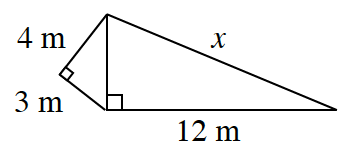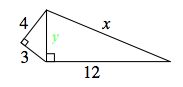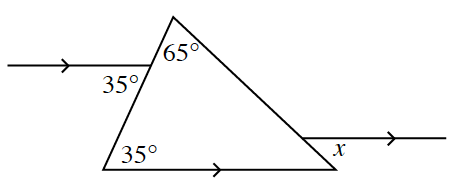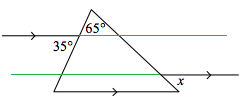### Home > INT2 > Chapter 5 > Lesson 5.2.4 > Problem5-104

5-104.

For each diagram below, solve for $x$. Name the relationship(s) you used. Show all work.

1.Use the Pythagorean Theorem to solve for $y$.
$(4^2+3^2=y^2)$After solving for $y$, use the Pythagorean Theorem again to solve for $x$.

$(y^2+12^2=x^2)$

1.Extend the parallel lines that have already been drawn.Now establish a relationship between $x$ and one of the angles that has already been given.

Use the Math Notes boxes in Lessons 2.1.1 and 2.1.4 if you need help remembering angle pair relationships.